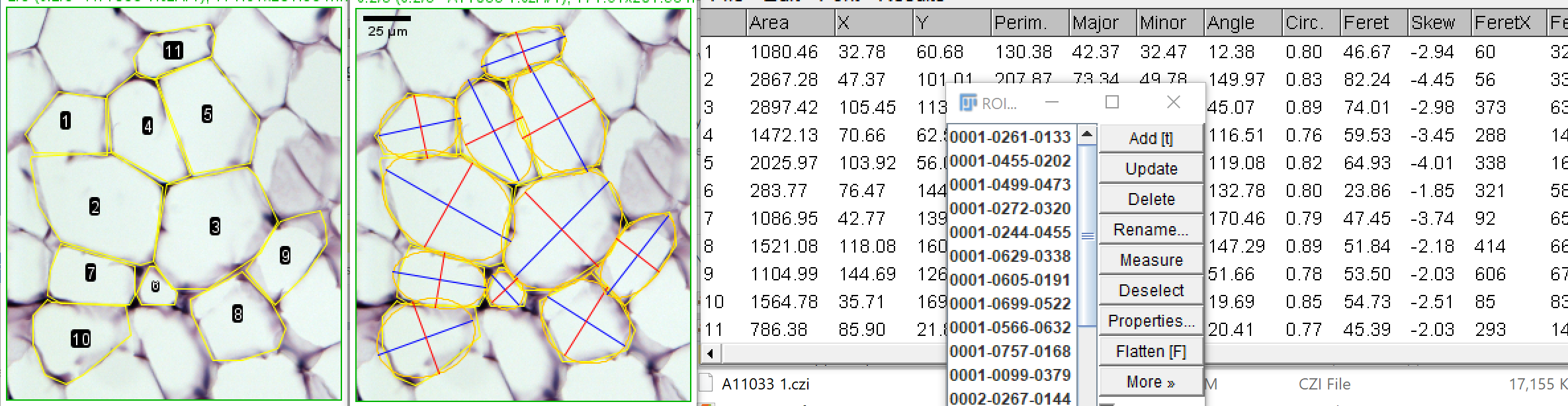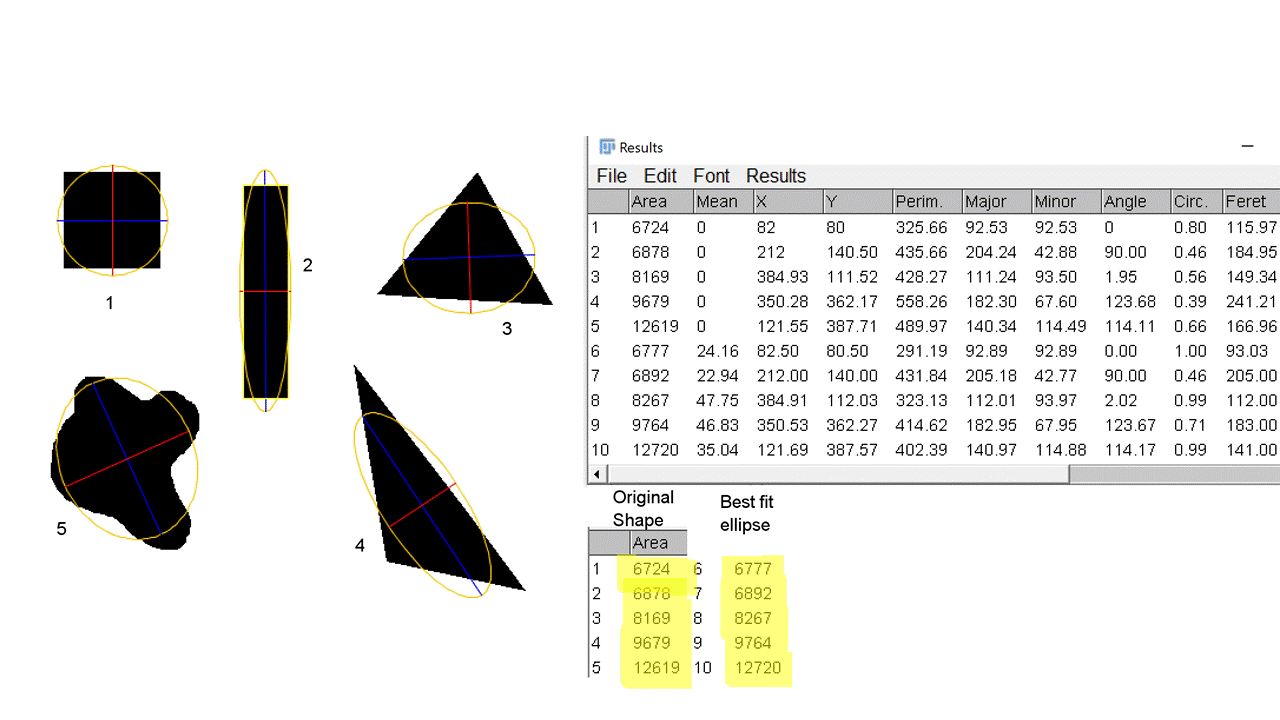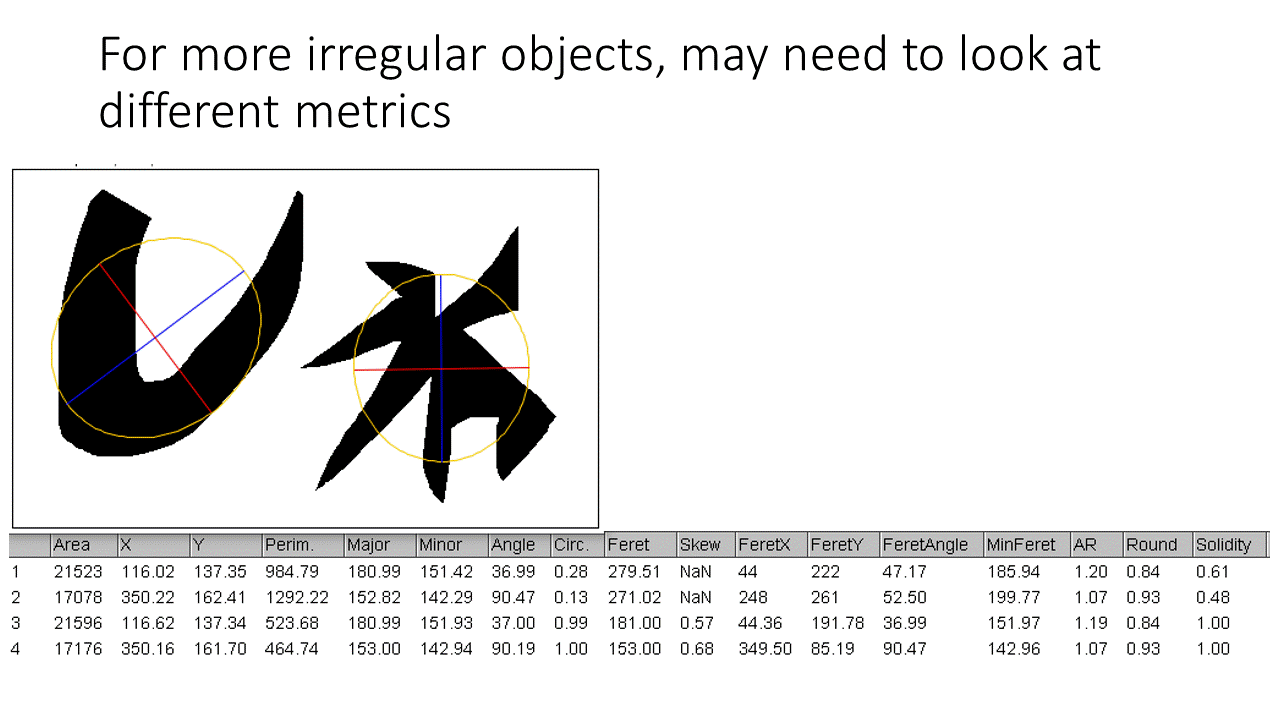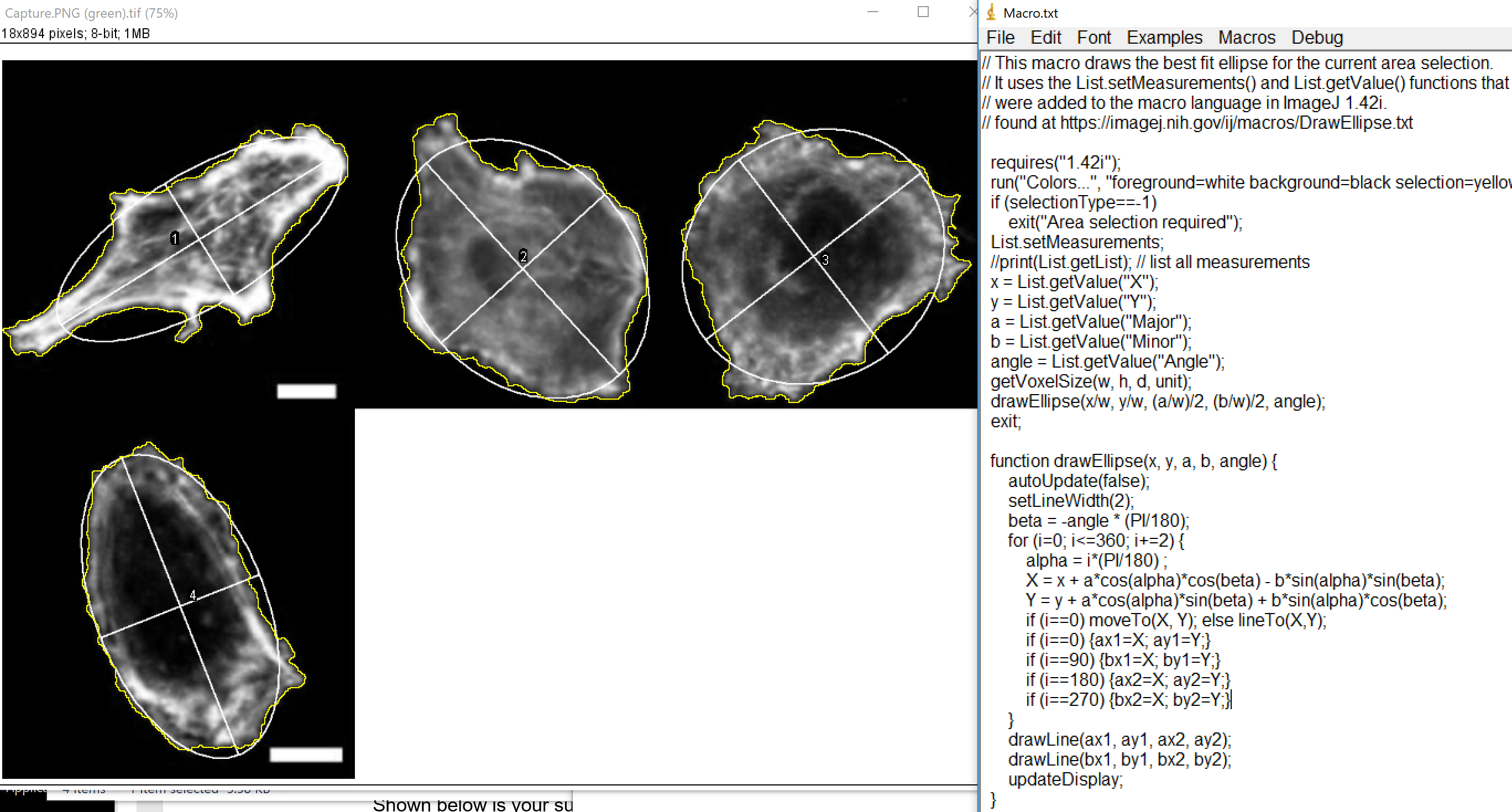Using ImageJ, are objects and their best fitting ellipse the same area?

First, let's look at the metric of best fitting ellipse. A common shape descriptor is also the ratio of the major and minor angle.Left: hand tracings for fat cells. The ROI Manager (right inset) contains these ROIs.
Clicking Measure button measured them all and put in Results table (right).
Middle: Best fitted ellipses with axes overlayed on image.

Now, to answer the question whether the ellipses have the same areas as the objects they represent.The answer is yes, approximately.This code on the ImageJ website draws the ellipses. I wrote different code to draw the ellipses (because so easy, two commands essentially) and pulled the axes code from somewhere else and changed it to use overlays.

/*	This macro draws best fitting ellipses.
* Needs to have open an image and a results window with X, Y, Major, Minor, angles measured.
* Practically, Area would also be relevant, but not needed for this macro.
* To get to this point, an image would either be thresholded and Analyze Particles would have
* populated ROI Manager with ROIs or manual tracings would be in ROI Manager.
*/
macro "Draw Best Fitting Ellipses" {
// requires run("Set Measurements...", "area centroid perimeter fit shape feret's redirect=None decimal=2");
original = getImageID;
originalTitle = getTitleStripExtension();
rename(originalTitle);
getVoxelSize(rescale, height, depth, unit);
for(i=0; i<nResults; i++) {
// draw ellipse
xc = getResult("X", i) / rescale;
yc = getResult("Y", i) / rescale;
major = getResult("Major", i) / rescale;
minor = getResult("Minor", i) / rescale;
angle = getResult("Angle", i);
makeOval(xc-(major/2), yc-(minor/2), major, minor);
run("Rotate...", " angle="+(180-angle));
roiManager("Add"); // comment out if don't want ellipses added to ROI Manager
run("Overlay Options...", "stroke=orange width=0 fill=none");
// draw axes
a = angle*PI/180; // convert angle degrees to radians
run("Overlay Options...", "stroke=blue width=0 fill=none");
d = major;
makeLine(xc+(d/2)*cos(a), yc-(d/2)*sin(a), xc-(d/2)*cos(a), yc+(d/2)*sin(a));
d=getResult('Minor',i);
a=a+PI/2; // rotate angle 90 degrees
run("Overlay Options...", "stroke=red width=0 fill=none");
d = minor;
makeLine(xc+(d/2)*cos(a), yc-(d/2)*sin(a), xc-(d/2)*cos(a), yc+(d/2)*sin(a));
}
run("Select None");
}

An earlier implementation (not written by me).

alternate_best_fit_ellipse_macro.txtFirst written 20020718 - last updated 20201008